Ex 4.3

Chapter 4 Class 8 Practical Geometry
Serial order wise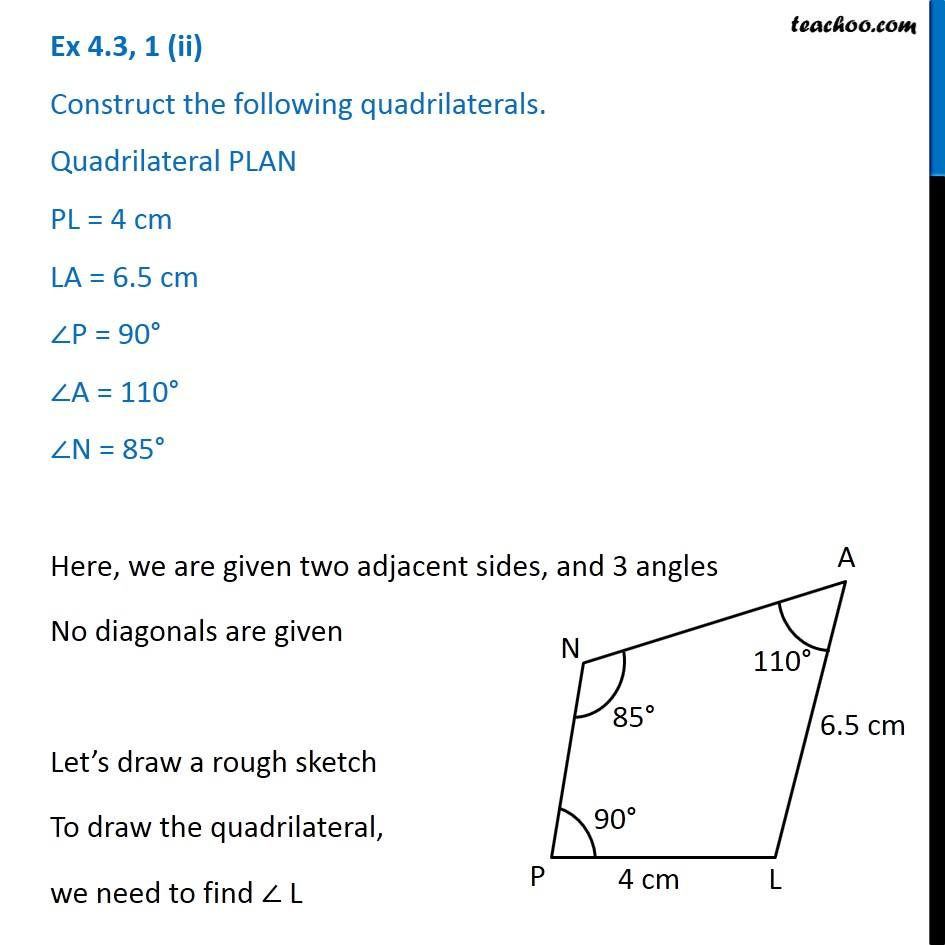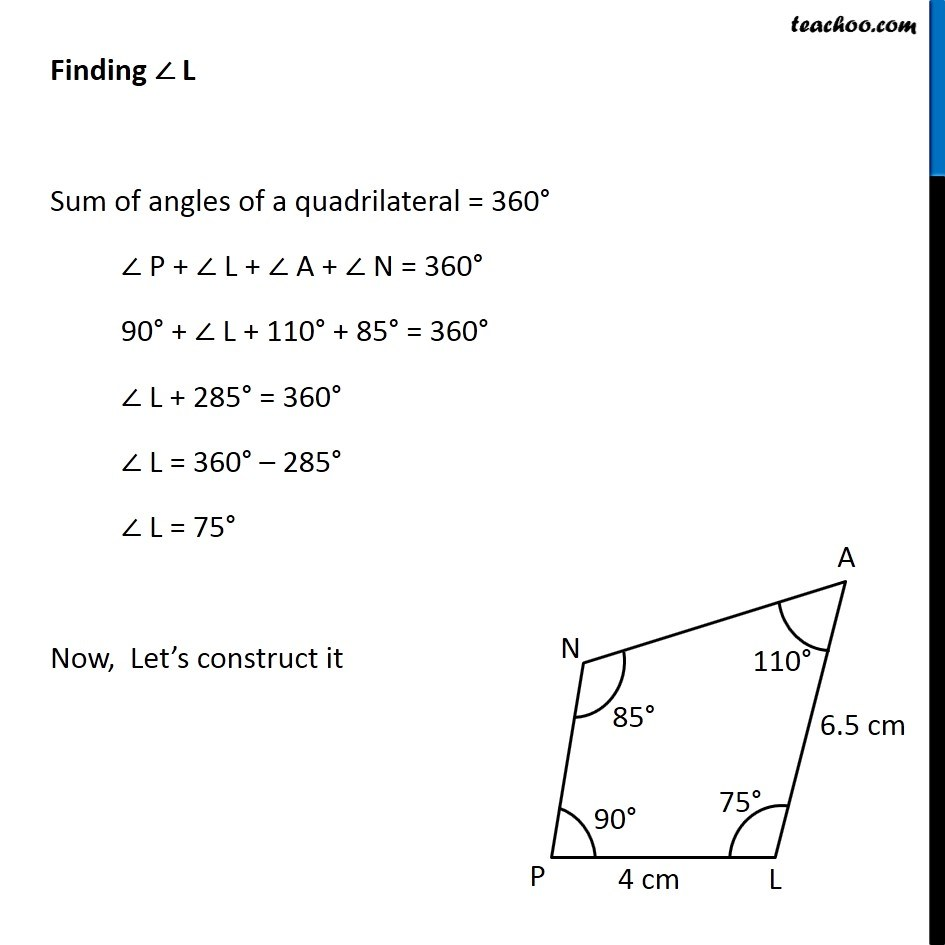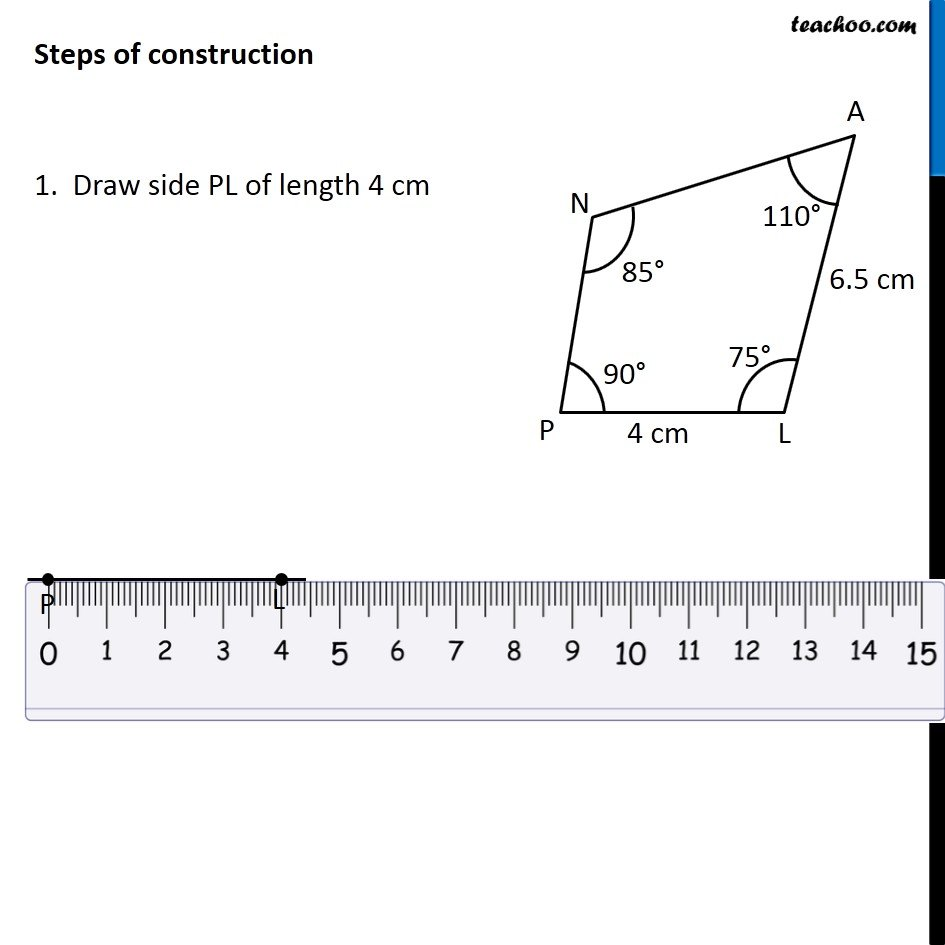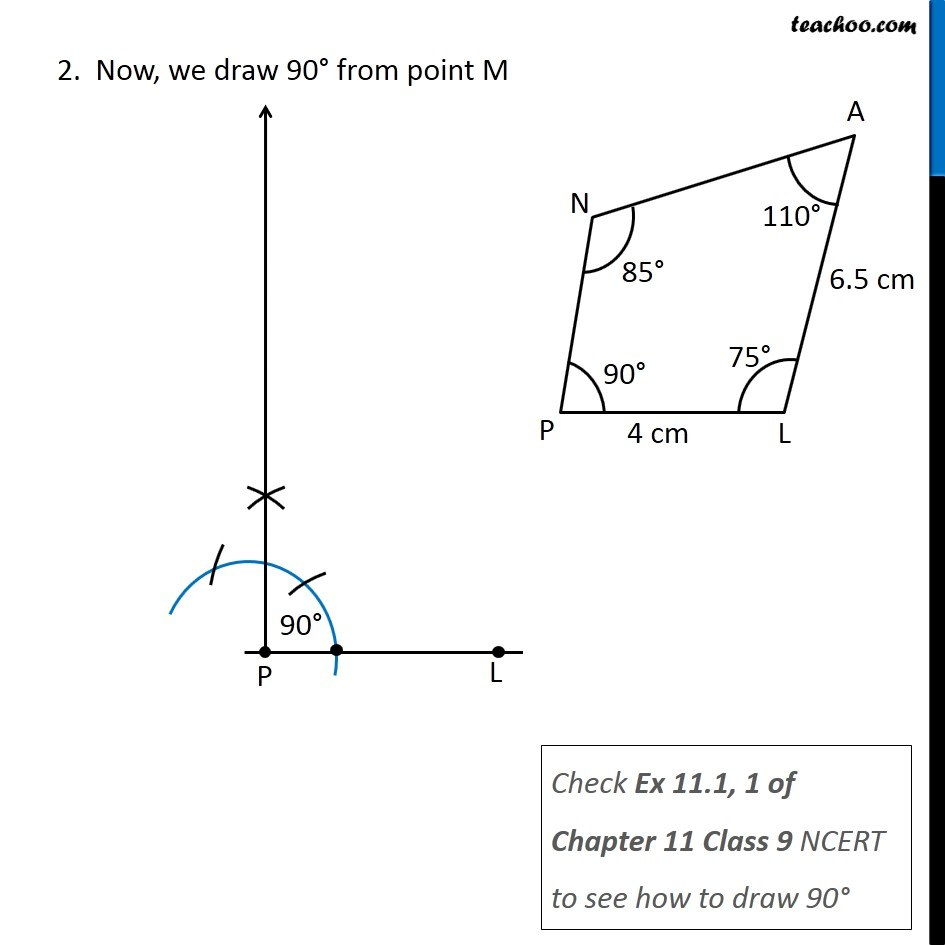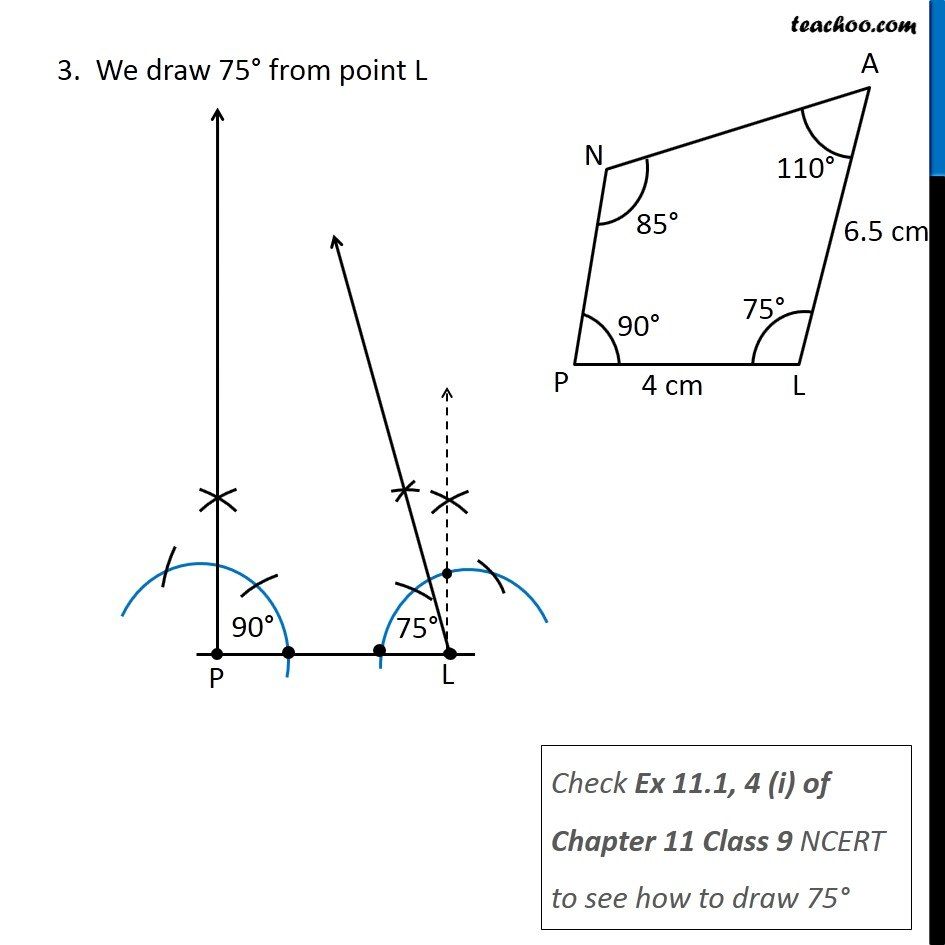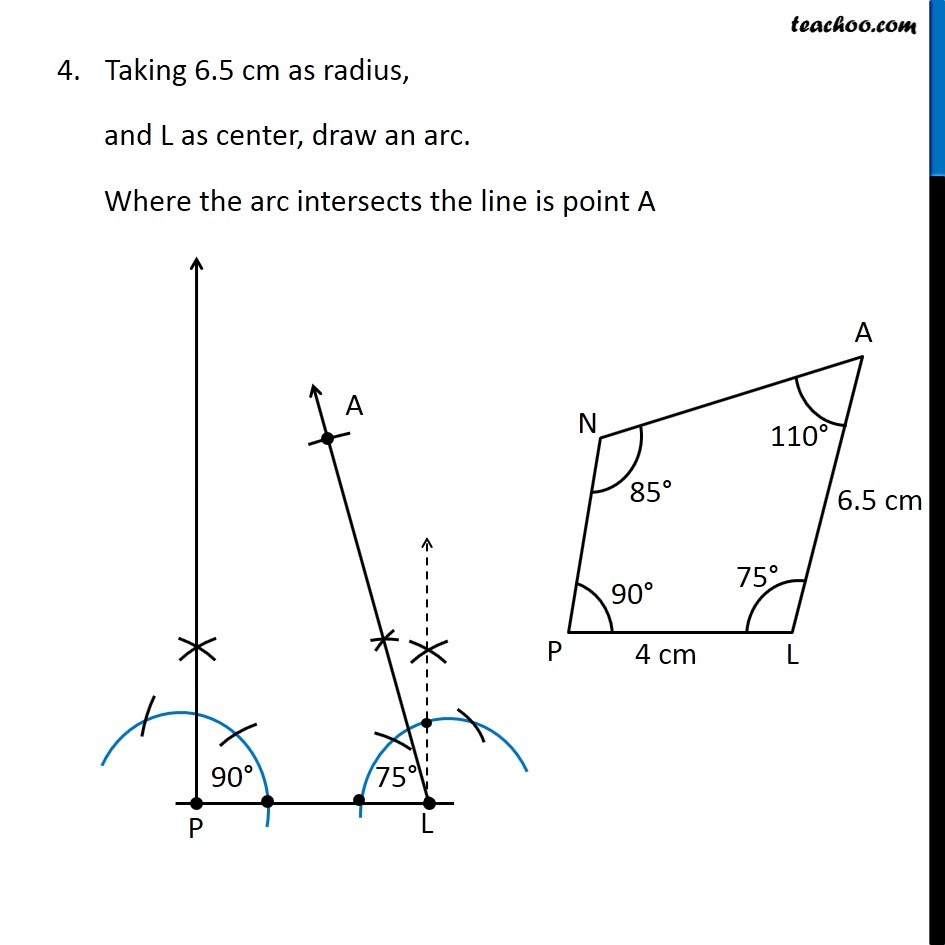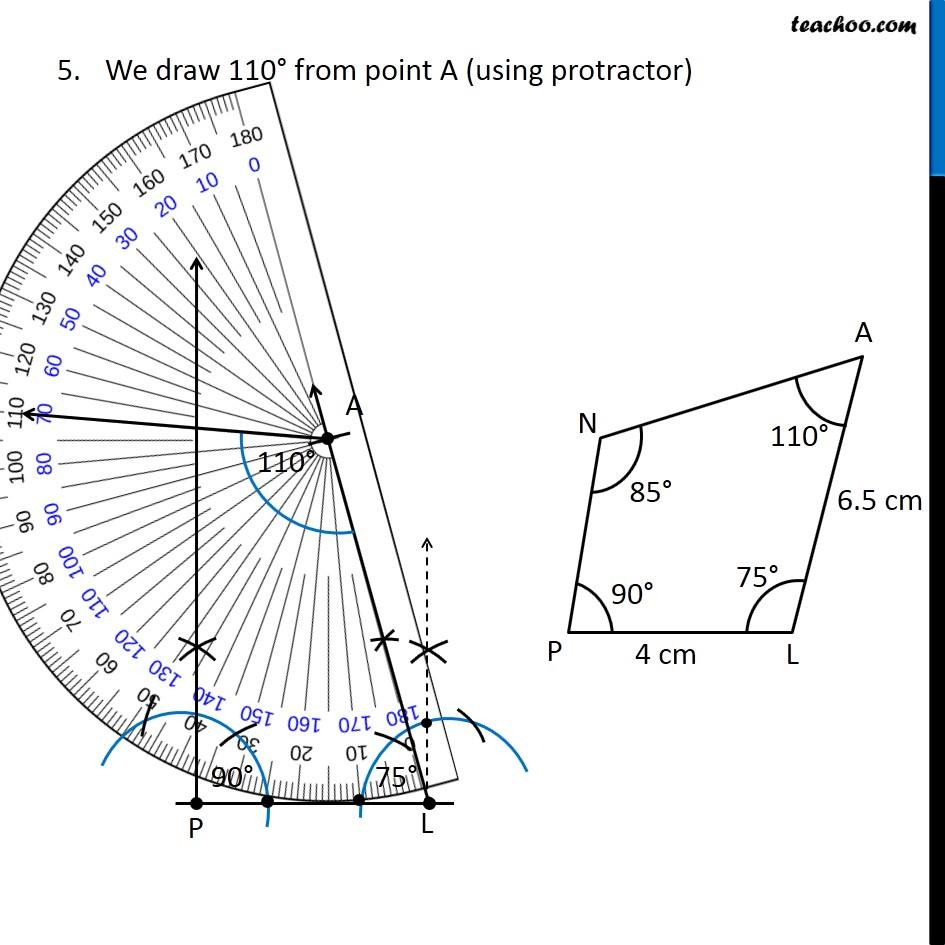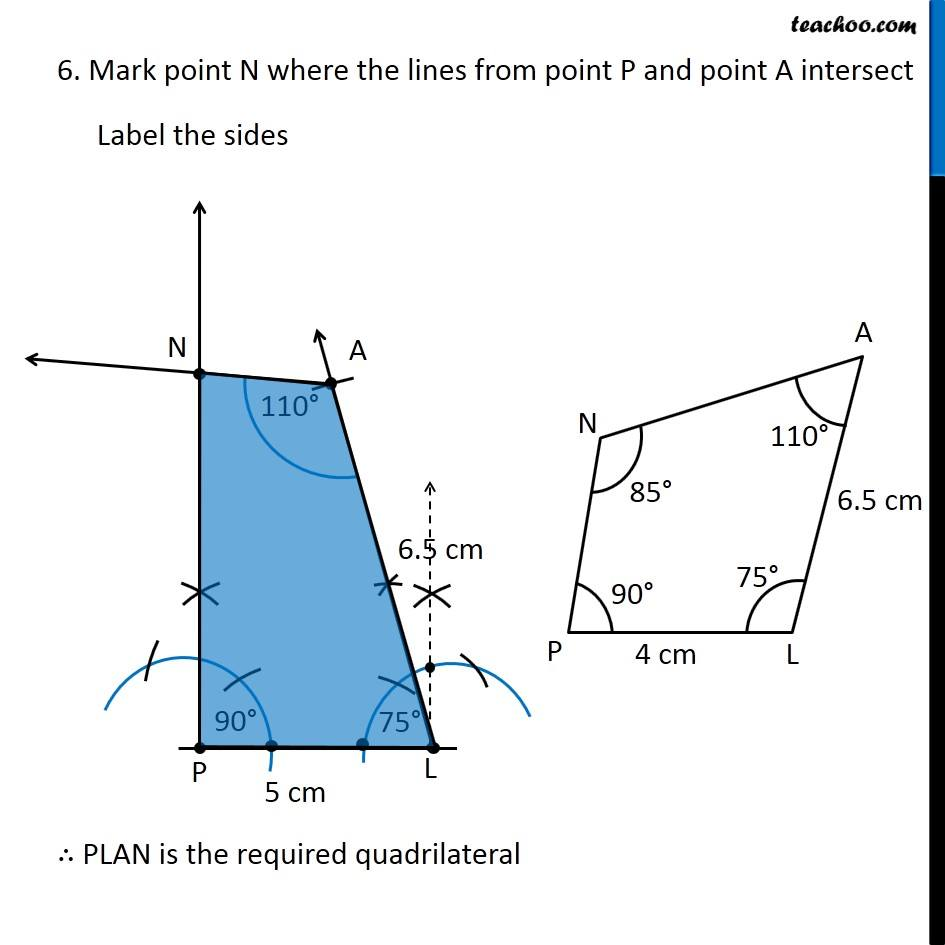Get live Maths 1-on-1 Classs - Class 6 to 12

### Transcript

Ex 4.3, 1 (ii) Construct the following quadrilaterals. Quadrilateral PLAN PL = 4 cm LA = 6.5 cm ∠P = 90° ∠A = 110° ∠N = 85° Here, we are given two adjacent sides, and 3 angles No diagonals are given Let’s draw a rough sketch To draw the quadrilateral, we need to find ∠ L Finding ∠ L Sum of angles of a quadrilateral = 360° ∠ P + ∠ L + ∠ A + ∠ N = 360° 90° + ∠ L + 110° + 85° = 360° ∠ L + 285° = 360° ∠ L = 360° – 285° ∠ L = 75° Now, Let’s construct it Steps of construction 1. Draw side PL of length 4 cm 2. Now, we draw 90° from point Check Ex 11.1, 1 of Chapter 11 Class 9 NCERT to see how to draw 90° 2. Now, we draw 90° from point M Check Ex 11.1, 1 of Chapter 11 Class 9 NCERT to see how to draw 90° Check Ex 11.1, 1 of Chapter 11 Class 9 NCERT to see how to draw 90° Check Ex 11.1, 4 (i) of Chapter 11 Class 9 NCERT to see how to draw 75° Taking 6.5 cm as radius, and L as center, draw an arc. Where the arc intersects the line is point A We draw 110° from point A (using protractor) 6. Mark point N where the lines from point P and point A intersect Label the sides ∴ PLAN is the required quadrilateral